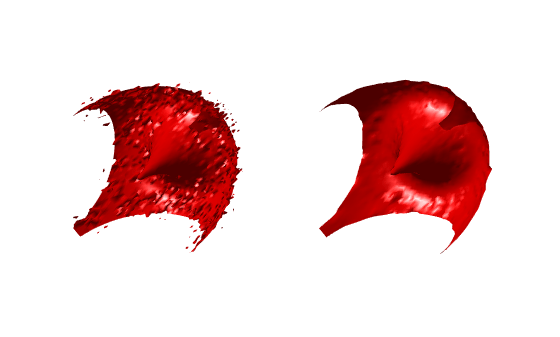# medfilt3

3-D median filtering

## Syntax

``B = medfilt3(A)``
``````B = medfilt3(A,[m n p])``````
``B = medfilt3(___,padopt)``

## Description

example

````B = medfilt3(A)` filters the 3-D image `A` with a 3-by-3-by-3 filter. By default, `medfilt3` pads the image by replicating the values in a mirrored way at the borders.```
``````B = medfilt3(A,[m n p])``` performs median filtering of the 3-D image `A` in three dimensions. Each output voxel in `B` contains the median value in the m-by-n-by-p neighborhood around the corresponding voxel in `A`. ```
````B = medfilt3(___,padopt)` controls how `medfilt3` pads the array boundaries.```

## Examples

collapse all

Create a noisy 3-D surface.

```[x,y,z,V] = flow(50); noisyV = V + 0.1*double(rand(size(V))>0.95) - 0.1*double(rand(size(V))<0.05);```

Apply median filtering.

`filteredV = medfilt3(noisyV);`

Display the noisy and filtered surfaces together.

```subplot(1,2,1) hpatch1 = patch(isosurface(x,y,z,noisyV,0)); isonormals(x,y,z,noisyV,hpatch1) set(hpatch1,'FaceColor','red','EdgeColor','none') daspect([1,4,4]) view([-65,20]) axis tight off camlight left lighting phong subplot(1,2,2) hpatch2 = patch(isosurface(x,y,z,filteredV,0)); isonormals(x,y,z,filteredV,hpatch2) set(hpatch2,'FaceColor','red','EdgeColor','none') daspect([1,4,4]) view([-65,20]) axis tight off camlight left lighting phong```## Input Arguments

collapse all

Input image, specified as a 3-D numeric or logical array.

Data Types: `single` | `double` | `int8` | `int16` | `int32` | `int64` | `uint8` | `uint16` | `uint32` | `uint64` | `logical`

Neighborhood size, specified as a 3-element vector of positive odd integers.

Data Types: `single` | `double` | `int8` | `int16` | `int32` | `uint8` | `uint16` | `uint32`

Padding option, specified as one of the following values:

ValueDescription
`'symmetric'`Pad array with mirror reflections of itself
`'replicate'`Pad array by repeating border elements
`'zeros'`Pad array with `0`s

Data Types: `char` | `string`

## Output Arguments

collapse all

Output image, returned as a 3-D numeric array of the same class and size as the input image `A`.